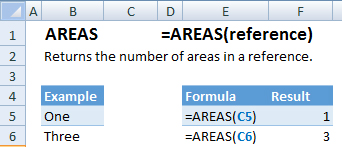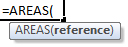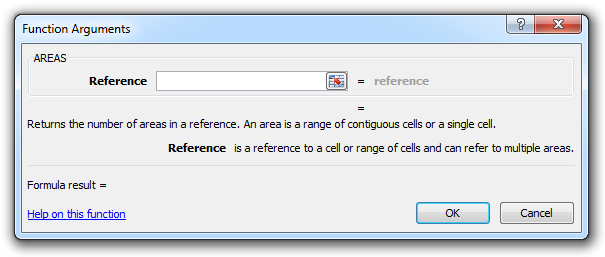### AREAS Function Examples – Excel & Google Sheets

This Excel Tutorial demonstrates how to use the Excel AREAS Function in Excel to calculate the number of areas in a reference, with formula examples.

## AREAS Function Description:

The AREAS Function Returns the number of areas in a reference.

## Formula Examples:Example Formula Result
One =AREAS(C5) 1
Three =AREAS(C6) 3
AutoMacro - VBA Code Generator

## Syntax and Arguments:

The Syntax for the AREAS Formula is:

`=AREAS(reference)`

### Function Arguments ( Inputs ):

reference – Cell reference or array of cells.

## Additional Notes

Use the AREAS Function to return the number of areas in a reference. The input may include more than one reference. When including more than one reference,You must include an extra set of parenthesis otherwise the function will generate an error.

Return to the List of all Functions in Excel

## How to use the AREAS Function in Excel:

To use the AND Excel Worksheet Function, type the following into a cell:
`=AND(`
After entering it in the cell, notice how the AND formula inputs appear below the cell:You will need to enter these inputs into the function. The function inputs are covered in more detail in the next section. However, if you ever need more help with the function, after typing “=AREAS(” into a cell, without leaving the cell, use the shortcut CTRL + A (A for Arguments) to open the “Insert Function Dialog Box” for detailed instructions:For more information about the AREAS Formula visit the
Microsoft Website.

## Excel Practice Worksheet

Practice Excel functions and formulas with our 100% free practice worksheets!

• Automatically Graded Exercises
• Learn Excel, Inside Excel!